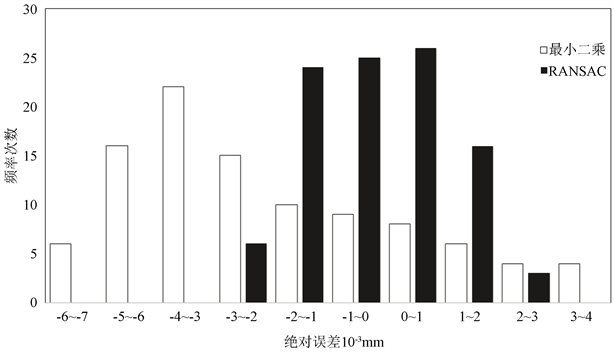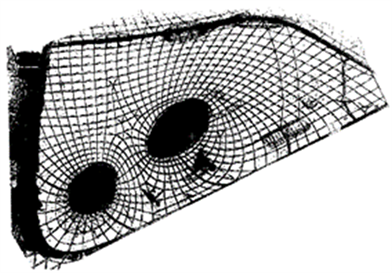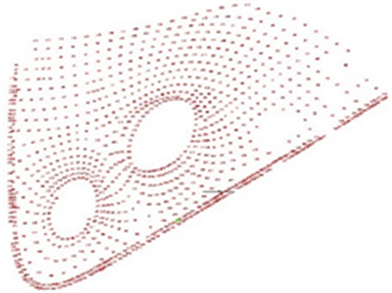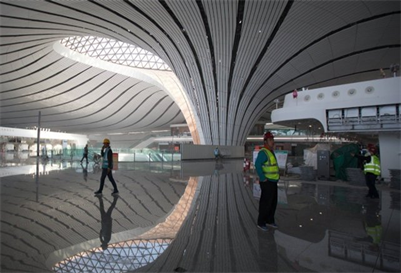# 基于三维激光点云的钢架螺栓球体节点要素提取研究Research on Geometric Element Extraction of Steel Frame Bolts Based on 3D Laser Point Cloud

• 全文下载: PDF(2364KB)    PP.195-203   DOI: 10.12677/GST.2019.74026
• 下载量: 212  浏览量: 301   科研立项经费支持

At present, in the construction of large-span and complex buildings such as new airports and high-speed railway stations, the main frame is usually constructed by steel frame structure, and the internal construction of such steel frame structures needs to obtain the geometric elements of the center point coordinates and diameter of the bolt sphere. In order to extract the geometrical elements of the bolt sphere efficiently and accurately, this paper obtains the point cloud data based on the 3D laser scanning technology, and proposes to extract the parameters by using the random sampling consensus method. The results of the random sampling consistency method and the least squares fitting result are compared. The random sampling consensus algorithm extracts the sphere information better and has higher precision.

1. 引言

2. 原理与方法

2.1. 最小二乘法

${\left(x-a\right)}^{2}+{\left(y-b\right)}^{2}+{\left(z-c\right)}^{2}={r}^{2}$ (1)

${x}^{2}+{y}^{2}+{z}^{2}-2ax-2by-2cz+{a}^{2}+{b}^{2}+{c}^{2}={r}^{2}$ (2)

${x}^{2}+{y}^{2}+{z}^{2}-Ax-By-Cz+D=0$ (3)

$a=\frac{A}{2},\text{\hspace{0.17em}}\text{\hspace{0.17em}}b=\frac{B}{2},\text{\hspace{0.17em}}\text{\hspace{0.17em}}c=\frac{C}{2},\text{\hspace{0.17em}}\text{\hspace{0.17em}}r=\sqrt{{a}^{2}+{b}^{2}+{c}^{2}-D}$ (4)

2.2. 随机采样算法Table 1. Random sampling algorithm parameters

N维球体被定义为满足以下公式的一组点：

${\left(p-c\right)}^{\text{T}}\left(p-c\right)={r}^{2}$ (5)

$\forall i,j\text{\hspace{0.17em}}\text{\hspace{0.17em}}\text{\hspace{0.17em}}{\left({p}_{i}-c\right)}^{\text{T}}\left({p}_{i}-c\right)={\left({p}_{j}-c\right)}^{\text{T}}\left({p}_{j}-c\right)={r}^{2}$ (6)

${\left({p}_{i}-{p}_{j}\right)}^{\text{T}}c=0.5\left({p}_{i}^{\text{T}}{p}_{i}-{p}_{j}^{\text{T}}{p}_{j}\right)$ (7)

$\left[\begin{array}{cc}\left({p}_{1x}-{p}_{2x}\right)& \left({p}_{1y}-{p}_{2y}\right)\\ \left({p}_{1x}-{p}_{3x}\right)& \left({p}_{1y}-{p}_{3y}\right)\end{array}\right]\left[\begin{array}{c}{c}_{x}\\ {c}_{y}\end{array}\right]=0.5\left[\begin{array}{c}\left({p}_{1x}^{2}-{p}_{2x}^{2}\right)+\left({p}_{1x}^{2}-{p}_{2y}^{2}\right)\\ \left({p}_{1x}^{2}-{p}_{3x}^{2}\right)+\left({p}_{1x}^{2}-{p}_{3y}^{2}\right)\end{array}\right]$ (8)

$A=\left(\begin{array}{cc}a& b\\ c& d\end{array}\right)$ , ${A}^{-1}=\frac{1}{ad-bc}\left[\begin{array}{cc}d& -b\\ -c& a\end{array}\right]$ (9)(10)

$A=\left[\begin{array}{ccc}a& b& c\\ d& e& f\\ g& h& i\end{array}\right],\text{\hspace{0.17em}}\text{\hspace{0.17em}}{A}^{-1}=\frac{1}{a\left(ei-fh\right)-b\left(di-gh\right)+c\left(dh-eg\right)}\left[\begin{array}{ccc}ei-fh& ch-bi& bf-ce\\ fg-di& ai-cg& cd-af\\ dh-eg& bg-ah& ae-ah\end{array}\right]$ (11)

${\delta }_{i}={p}_{i}^{\text{T}}{p}_{i}-2{p}_{i}^{\text{T}}c+{c}^{\text{T}}c-{r}^{2}$ (12)

$\left[{c}^{*},{r}^{*}\right]=\mathrm{arg}{\mathrm{min}}_{c,r}{\sum }_{i=1}^{m}{\delta }_{i}{}^{2}$ (13)

$m={c}^{\text{T}}c-{r}^{2}$，得到以下线性方程组 $\left(Ax=b\right)$(14)

${\sum }_{i=1}^{m}{\delta }_{i}{}^{2}={‖Ax-b‖}^{2}$ (15)

3. 实验与分析

“全站仪 + 最小二乘法”是利用全站仪采集螺栓球体表面上的4个随机点，然后通过MATLAB编写的最小二乘法程序对数据进行计算，最终得到螺栓球的半径和球体中心坐标。Figure 1. Experimental flowTable 2. RANSAC processing results (unit: m)Table 3. Least squares processing results (unit: m)Figure 2. Error statistics for two experimental methods

4. 实际应用Figure 3. International airport point cloudFigure 4. Bolt ball lowest point coordinatesFigure 5. Construction site before ceiling installationFigure 6. Site after completion of ceiling ceiling work

5. 结论

  戴升山, 李田凤. 地面三维激光扫描技术的发展与应用前景[J]. 现代测绘, 2009, 32(4): 11-12.  Lu, T.D., Zhou, S.J. and Zhang, L.T. (2009) Sphere Target Fixing of Point Cloud Data Based on TLS. Journal of Geodesy & Geodynamics, 7492, 296-299.  Yuan, B. (2013) Spherical Target Positioning of Terrestrial 3D Laser Scanning Based on Robust Weighted Total Least Squares Method. Site Investigation Science and Technology, 1, 19-22.  Lu, T.D. and Zhou, S.J. (2010) An Iterative Algorithm for Total Least Squares Estimation. Geomatics & Information Science of Wuhan University, 27, 106-108.  权力奥, 杨荣华, 潘蕾西兰, 等. 基于球体几何关系的球标靶特征提取算法[J]. 大地测量与地球动力学, 2017, 37(8) :860-863.  万建鹏, 叶素倩. 球面点云数据拟合的总体最小二乘解法[J]. 江西科学, 2017, 35(2): 197-199.  Yaniv, Z. (2010) Random Sample Consensus (RANSAC) Algorithm, A Generic Implementation. The Insight Journal.  杨必胜, 梁福逊, 黄荣刚. 三维激光扫描点云数据处理研究进展、挑战与趋势[J]. 测绘学报, 2017, 46(10): 311-318.